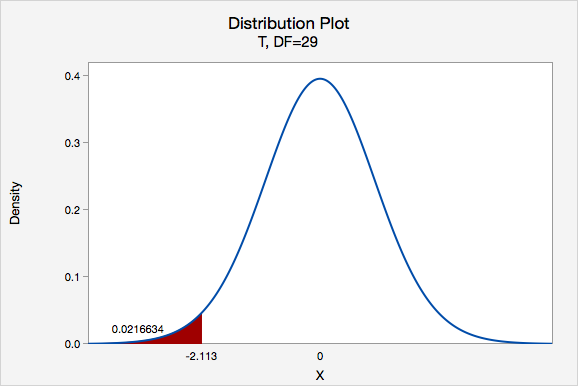# 8.2.3.1.4 - Example: Transportation Costs

8.2.3.1.4 - Example: Transportation Costs

According to CNN, in 2011, the average American spent \$16,803 on housing. A suburban community wants to know if their residents spent less than this national average. In a survey of 30 randomly selected residents, they found that they spent an annual average of \$15,800 with a standard deviation of \\$2,600.

1. Check assumptions and write hypotheses

Housing costs are quantitative. Because $$n \ge 30$$, the sampling distribution can be approximated using the $$t$$ distribution.

This is a left-tailed test because we want to know if residents of this community spent less than the national average.

$$H_{0}:\mu=16803$$
$$H_{a}:\mu<16803$$

2. Calculate the test statistic
Test Statistic: One Group Mean

$$t=\dfrac{\overline{x}-\mu_0}{\dfrac{s}{\sqrt{n}}}$$

$$\overline{x}$$ = sample mean
$$\mu_{0}$$ = hypothesized population mean
$$s$$ = sample standard deviation
$$n$$ = sample size

$$t=\dfrac{\overline{x}-\mu_0}{\dfrac{s}{\sqrt{n}}}=\dfrac{15800-16803}{\dfrac{2600}{\sqrt{30}}}=-2.113$$

Our t test statistics is -2.113

3. Determine the p-value

$$df=n-1=30-1=29$$

This is a left-tailed test so we want to know the probability of $$t < -2.113$$Using Minitab we can find that $$p=0.0216634$$

4. Make a decision

$$p\leq .05$$, therefore we reject the null hypothesis.

5. State a "real world" conclusion

There is evidence to state that on average residents of this community spent less than the national average on housing in 2011.

  Link ↥ Has Tooltip/Popover Toggleable Visibility